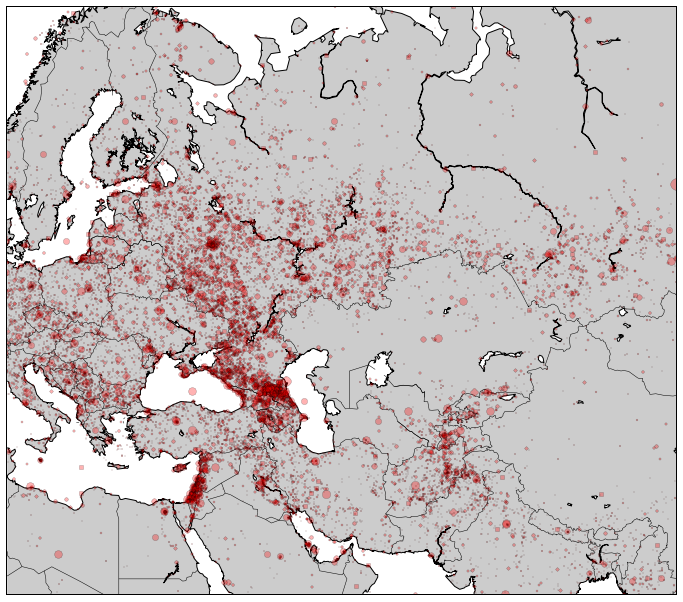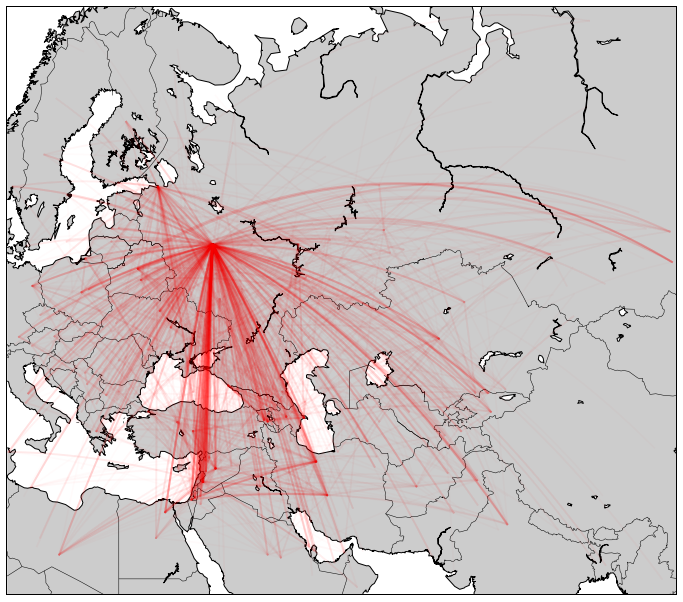# Mapping with GDELT¶

Department of Computational Social Science, George Mason University

What's better than awesome data? Awesome data on a map. I loved Rolf Fredheim's tutorial on mapping GDELT in R, so I decided to try and replicate (some of) it in Python.

For the basics of working with GDELT, check out my previous tutorial, Rolf's, or John Beieler's.

In :
# Some code to style the IPython notebook and make it more legible.
# https://github.com/CamDavidsonPilon/Probabilistic-Programming-and-Bayesian-Methods-for-Hackers
from IPython.core.display import HTML
HTML(styles)

Out:

The key Python package you need for this is the Basemap toolkit for Matplotlib. I found it to be non-trivial to set up on my Mac. Before you can install it, you need the GEOS library. The Mac binaries helped me, but your mileage may vary.

(Here's one advantage that R has over Python; the packages seem to work much easier out of the box, and you get slightly prettier maps at the end.)

In :
import datetime as dt
from collections import defaultdict

import matplotlib.pyplot as plt

from mpl_toolkits.basemap import Basemap

In :
# Set this variable to the directory where the GDELT data files are
PATH = "GDELT.1979-2012.reduced/"


We quickly refresh ourselves on the column names and indices; for this analysis, we care about the geolocation data: the lat and long coordinates for each actor, and for the event itself.

In :
with open(PATH+"2010.reduced.txt") as f:
for i, col_name in enumerate(col_names):
print i, col_name

0 Day
1 Actor1Code
2 Actor2Code
3 EventCode
5 GoldsteinScale
6 Actor1Geo_Lat
7 Actor1Geo_Long
8 Actor2Geo_Lat
9 Actor2Geo_Long
10 ActionGeo_Lat
11 ActionGeo_Long



For the first pass, we grab all the rows of data that involve any Russian actors (country code RUS).

In :
data = []
for year in range(1979, 2013):
f = open(PATH + str(year) + ".reduced.txt")
for raw_row in f:
row = raw_row.split("\t")
actor1 = row[:3]
actor2 = row[:3]
both = actor1 + actor2
if "RUS" in both:
data.append(raw_row)
print "Russia-related records:", len(data)

Russia-related records: 3928349


Next, we iterate through all the rows and count the events by coordinates. We'll use a defaultdict keyed on a (lat, long) tuple to store the counts. Then we'll run some basic summary statistics on the counts to get an idea for the distribution; are there many points with roughly similar event counts, or a few with high event counts and many with low ones?

In :
point_counts = defaultdict(int) # Defaultdict with (lat, long) as key
for row in data:
row = row.split("\t")
try:
lat = float(row)
lon = float(row)
point_counts[(lat, lon)] += 1
except:
pass

# Get some summary statistics
counts = np.array(point_counts.values())
print "Total points:", len(counts)
print "Min events:", counts.min()
print "Max events:", counts.max()
print "Mean events:", counts.mean()
print "Median points:", np.median(counts)

Total points: 52603
Min events: 1
Max events: 1086076
Mean events: 73.4356215425
Median points: 2.0


These numbers suggest a distribution with very high variance; the number of events at most points are small, but some get very, very large. In fact, we might be dealing with a power law. Work with social-scientific or complex data for any length of time, and you'll start seeing power laws everywhere. There are some good reasons for this, but we don't need to worry about them in order to make a cool map.

So why do we care about the distribution right now? Because we need to know how to plot the points. Make the smallest ones too small, and we won't be able to see them; make the largest ones too large, and it will overwhelm the rest of the map. A log-scale is probably a good call here; a point with 10x as many events will be twice the size on our map.

Putting data on maps is quite literally more art than science. It took me some tweaking to find something that looked good. I ended up settling on taking the log of the event count for each point + 1 (since $log(1) = 0$ ), and multiplying it by 2 to get a size in points. Take my word for it, or play around with it to make it better.

In :
def get_size(count):
'''
Convert a count to a point size.
Log-scaled.
'''
scale_factor = 2
return np.log10(count + 1) * scale_factor


Now we need to draw the actual map. We do this in several steps; as I understand it, each step is drawing a different 'layer' onto our image.

First, we define a Basemap object. We pick a projection for it, choose a resolution (we should go with low, since we're looking at a big chunk of the world) and set a bounding box.

Once we have the Basemap, we call a few of its built-in methods to draw the coastlines, the country boundaries, fill the landmass with color, and draw the box around the map.

Finally, once we've done all that, we'll iterate over each of our points and draw them on the map, one at a time. Calling the map object itself will convert the lat and long coordinates to y and x on our map image (and remember that lat = y, long = x). The plot method will draw the point at the map itself. For each point, we'll use the get_size function above to convert the event count to a size. Finally, we'll fix point transparency (alpha) at 0.3: enough to see, without completely overwhelming the map, and providing a nice layering effect when many points cluster together. But again, more art than science; tweak it and see what looks good to you!

In :
# Note that we're drawing on a regular matplotlib figure, so we set the
# figure size just like we would any other.
plt.figure(figsize=(12,12))

# Create the Basemap
event_map = Basemap(projection='merc',
resolution='l', area_thresh=1000.0, # Low resolution
lat_0 = 55.0, lon_0=60.0, # Map center
llcrnrlon=10, llcrnrlat=20, # Lower left corner
urcrnrlon=100, urcrnrlat=70) # Upper right corner

# Draw important features
event_map.drawcoastlines()
event_map.drawcountries()
event_map.fillcontinents(color='0.8') # Light gray
event_map.drawmapboundary()

# Draw the points on the map:
for point, count in point_counts.iteritems():
x, y = event_map(point, point) # Convert lat, long to y,x
marker_size = get_size(count)
event_map.plot(x,y, 'ro', markersize=marker_size, alpha=0.3)Next we'll map interactions; to keep things manageable and reduce map clutter, we'll only look at 2012 events. For each event, we get the lat and long coordinates for Actor 1 (columns 6,7) and Actor 2 (columns 8,9).

We'll count again using a defaultdict, but this time the keys will be pairs of points.

In :
# Defaultdict with ((lat, long), (lat,long)) as key
interaction_counts = defaultdict(int)

for row in data:
row = row.split("\t")
# Skip row if not in 2012
if row[:4] != '2012':
continue
try:
lat_1 = float(row)
lon_1 = float(row)
lat_2 = float(row)
lon_2 = float(row)

interaction_counts[((lat_1, lon_1), (lat_2, lon_2))] += 1
except:
pass
# Check point data:
counts = np.array(interaction_counts.values())
print "Total point-pairs:", len(counts)
print "Min events:", counts.min()
print "Max events:", counts.max()
print "Mean events:", counts.mean()
print "Median points:", np.median(counts)

Total point-pairs: 27785
Min events: 1
Max events: 34160
Mean events: 7.24218103293
Median points: 1.0


Instead of points, here we'll be drawing lines between points. So instead of size, let's scale line transparency. Again, it took some tweaking to find a scaling that worked well, but I ended up settling on log-scaling relative to the maximum count (since transparency is always between 0 and 1), scaled by 0.25. Play around with it and see what works for you.

In :
max_val = np.log10(counts.max())

def get_alpha(count):
'''
Convert a count to an alpha val.
Log-scaled
'''
scale = np.log10(count)
return (scale/max_val) * 0.25


The first step of the map drawing will be the same. Create the Basemap and draw the key geographic features.

Next, we'll draw a line for each point. Specifically, we'll draw a great circle segment, which has a nice Basemap function, is the actual 'straight line' on a globe, and looks pretty cool to boot. Confusingly, unlike for drawing points, we don't need to convert lat/long coordinates to x/y in order to draw the great circle line.

In :
# Draw the basemap like before
plt.figure(figsize=(12,12))
event_map = Basemap(projection='merc',
resolution='l', area_thresh=1000.0, # Low resolution
lat_0 = 55.0, lon_0=60.0, # Map center
llcrnrlon=10, llcrnrlat=20, # Lower left corner
urcrnrlon=100, urcrnrlat=70) # Upper right corner

# Draw important features
event_map.drawcoastlines()
event_map.drawcountries()
event_map.fillcontinents(color='0.8')
event_map.drawmapboundary()

# Draw the lines on the map:
for arc, count in interaction_counts.iteritems():
point1, point2 = arc
y1, x1 = point1
y2, x2 = point2

# Only plot lines where both points are on our map:
if ((x1 > 10 and x1 < 100 and y1 > 20 and y1 < 70) and
(x2 > 10 and x2 < 100 and y2 > 20 and y2 < 70)):

line_alpha = get_alpha(count)

# Draw the great circle line
event_map.drawgreatcircle(x1, y1, x2, y2, linewidth=2,
color='r', alpha=line_alpha)So there you have some cool visualizations with GDELT. Of course, pretty pictures != actual analysis. But sometimes visualizations can help kickstart your analysis by showing you things you might not have noticed otherwise. And once you have good analysis, visualizations can be one of the best ways to convey your point quickly and concisely.

So what are you waiting for? Get to work!